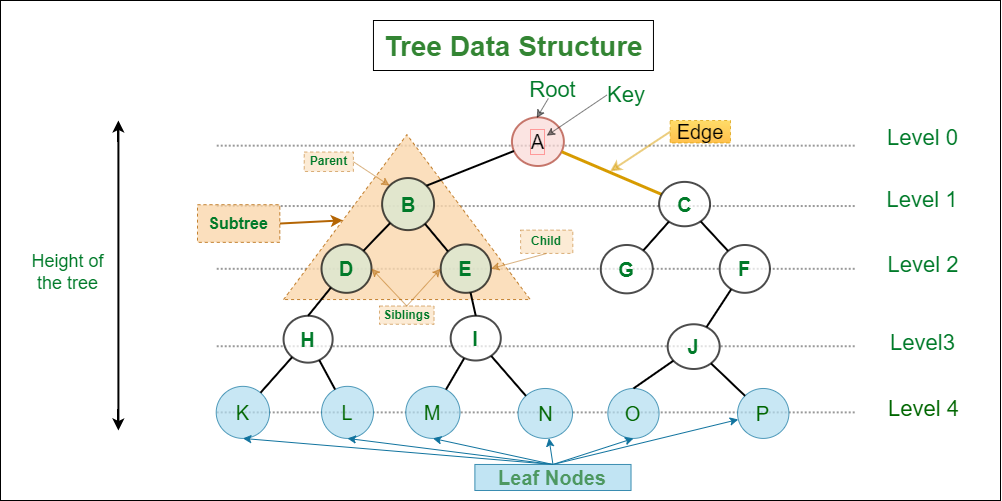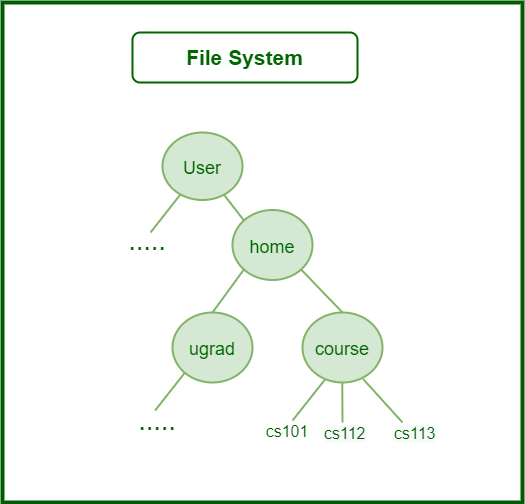# Applications of tree data structureApplications of tree data structure

## What is Tree Data Structure?

A tree is a type of data structure that represents a hierarchical relationship between data elements, called nodes. The top node in the tree is called the root, and the elements below the root are called child nodes. Each child node may have one or more child nodes of its own, forming a branching structure. The nodes at the bottom of the tree, which do not have any child nodes, are called leaf nodes.

A tree is a non-linear data structure, meaning that elements are not stored in a linear sequence like in an array or a linked list. Instead, elements are organized in a hierarchical structure, with each element having a parent-child relationship with other elements.

A tree can be represented in many ways, such as in an array or a linked list, but the most common representation is a graphical one, where each node is represented as a circle and each edge is represented as a line connecting two circles.

## Terminologies used in Tree:

According to the above example image of a tree

• Nodes: A B C D E F G H I J K L M N O P
• Root: A
• Internal Nodes: A B C D E F H I J
• External nodes:  K L M N O P
• (Parent , Child) : (A, B and C), (B, D and E), (C, G and F),(H, K and L), (I, M and N) , (J, O AND P).
• Siblings : (B,C) , (D, E), (G, F), (K, L), (M, N) ,(O, P)

## Why Tree to use Data Structure?

Unlike Array and Linked List, which are linear data structures, tree is hierarchical (or non-linear) data structure.• Hierarchical Structure: Trees are used to model hierarchical structures, such as the file system in a computer or the organizational chart in a company. The tree structure allows for a natural representation of parent-child relationships, making it easy to understand and visualize the data.
• Searching Efficiency: Trees provide an efficient way to search for data. For example, in a binary search tree, searching for a value takes time proportional to the logarithm of the number of elements, which is much faster than searching in a linear data structure like an array or a linked list.
• Sorting: Trees can be used to sort data efficiently. For example, in a self-balancing binary search tree, the data is automatically sorted as it is inserted into the tree, making it easy to find the minimum, maximum, and other values in the tree.
• Dynamic Data: Trees are dynamic data structures, which means that they can grow and shrink as needed. This makes them well-suited for applications where the data changes frequently, such as in real-time systems.
• Efficient Insertion and Deletion: Trees provide efficient algorithms for inserting and deleting data, which is important in many applications where data needs to be added or removed frequently.
• Easy to Implement: Trees are relatively easy to implement, especially when compared to other data structures like graphs. This makes them a popular choice for many programming projects.

## Other Applications of Tree Data Structure:

1. Store hierarchical data, like folder structure, organization structure, XML/HTML data.
2. Binary Search Tree is a tree that allows fast search, insert, delete on a sorted data. It also allows finding closest item
3. Heap is a tree data structure which is implemented using arrays and used to implement priority queues.
4. B-Tree and B+ Tree : They are used to implement indexing in databases.
5. Syntax Tree:  Scanning, parsing , generation of code and evaluation of arithmetic expressions in Compiler design.
6. K-D Tree: A space partitioning tree used to organize points in K dimensional space.
7. Trie : Used to implement dictionaries with prefix lookup.
8. Suffix Tree : For quick pattern searching in a fixed text.
9. Spanning Trees and shortest path trees are used in routers and bridges respectively in computer networks
10. As a workflow for compositing digital images for visual effects.
11. Decision trees.
12. Organization chart of a large organization.
13. In XML parser.
14. Machine learning algorithm.
15. For indexing in database.
16. IN server like DNS (Domain Name Server)
17. In Computer Graphics.
18. To evaluate an expression.
19. In chess game to store defense moves of player.
20. In java virtual machine.
21. Tree data structures are used to organize and manage files and directories in a file system. Each file and directory is represented as a node in the tree, with parent-child relationships indicating the hierarchical structure of the file system.
22. Tree data structures are often used in parsing, such as in compilers and interpreters, to represent the structure of a program or a document.
23. Tree data structures, such as binary search trees, are commonly used to implement efficient searching and sorting algorithms.
24.  Graphics and UI design
25.  Tree data structures are commonly used in decision-making algorithms in artificial intelligence, such as game-playing algorithms, expert systems, and decision trees.
26. Tree data structures can be used to represent the topology of a network and to calculate routing tables for efficient data transmission.